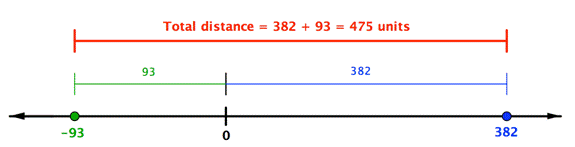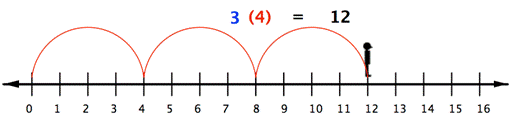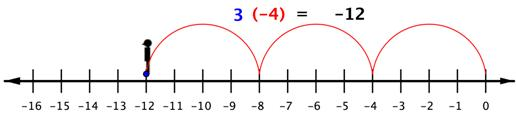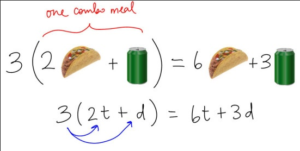## Real Numbers

### Learning Outcomes

• Add and subtract real numbers
• Add real numbers with the same and different signs
• Subtract real numbers with the same and different signs
• Simplify combinations that require both addition and subtraction of real numbers.
• Multiply and divide real numbers
• Multiply two or more real numbers.
• Divide real numbers
• Simplify expressions with both multiplication and division
• Simplify expressions with real numbers
• Recognize and combine like terms in an expression
• Simplify compound expressions with real numbers
• Simplify expressions with fraction bars, brackets, and parentheses
• Use the distributive property to simplify expressions with grouping symbols
• Simplify expressions containing absolute values

Some important terminology to remember before we begin is as follows:

• integers: counting numbers like 1, 2, 3, etc., including negatives and zero
• real number: fractions, negative numbers, decimals, integers, and zero are all real numbers
• absolute value: a number’s distance from zero; it’s always positive.  $|-7| = 7$
• sign: this refers to whether a number is positive or negative, we use $+$ for positive (to the right of zero on the number line) and $-$ for negative (to the left of zero on the number line)
• difference: the result of subtraction
• sum: the result of addition

The ability to work comfortably with negative numbers is essential to success in algebra. For this reason we will do a quick review of adding, subtracting, multiplying and dividing integers. Integers are all the positive whole numbers, zero, and their opposites (negatives). As this is intended to be a review of integers, the descriptions and examples will not be as detailed as a normal lesson.

## Adding and Subtracting Real Numbers

When adding integers we have two cases to consider. The first case is whether the signs match (both positive or both negative). If the signs match, we will add the numbers together and keep the sign.

If the signs don’t match (one positive and one negative number) we will subtract the numbers (as if they were all positive) and then use the sign from the larger number. This means if the larger number is positive, the answer is positive. If the larger number is negative, the answer is negative.

### To add two numbers with the same sign (both positive or both negative)

• Add their absolute values (without the $+$ or $-$ sign)
• Give the sum the same sign.

### To add two numbers with different signs (one positive and one negative)

• Find the difference of their absolute values. (Note that when you find the difference of the absolute values, you always subtract the lesser absolute value from the greater one.)
• Give the sum the same sign as the number with the greater absolute value.

### Example

Find $23–73$.

Another way to think about subtracting is to think about the distance between the two numbers on the number line. In the example below, $382$ is to the right of 0 by $382$ units, and $−93$ is to the left of 0 by 93 units. The distance between them is the sum of their distances to 0: $382+93$.### Example

Find $382–\left(−93\right)$.

The following video explains how to subtract two signed integers.

### Example

Find $-\frac{3}{7}-\frac{6}{7}+\frac{2}{7}$

In the following video you will see an example of how to add three fractions with a common denominator that have different signs.

### Example

Evaluate $27.832+(−3.06)$. When you add decimals, remember to line up the decimal points so you are adding tenths to tenths, hundredths to hundredths, and so on.

In the following video are examples of adding and subtracting decimals with different signs.

## Multiplying and Dividing Real Numbers

Multiplication and division are inverse operations, just as addition and subtraction are. You may recall that when you divide fractions, you multiply by the reciprocal. Inverse operations “undo” each other.

## Multiply Real Numbers

Multiplying real numbers is not that different from multiplying whole numbers and positive fractions. However, you haven’t learned what effect a negative sign has on the product.

With whole numbers, you can think of multiplication as repeated addition. Using the number line, you can make multiple jumps of a given size. For example, the following picture shows the product $3\cdot4$ as 3 jumps of 4 units each.So to multiply $3(−4)$, you can face left (toward the negative side) and make three “jumps” forward (in a negative direction).The product of a positive number and a negative number (or a negative and a positive) is negative.

### The Product of a Positive Number and a Negative Number

To multiply a positive number and a negative number, multiply their absolute values. The product is negative.

### Example

Find $−3.8(0.6)$.

The following video contains examples of how to multiply decimal numbers with different signs.

### The Product of Two Numbers with the Same Sign (both positive or both negative)

To multiply two positive numbers, multiply their absolute values. The product is positive.

To multiply two negative numbers, multiply their absolute values. The product is positive.

### Example

Find $~\left( -\frac{3}{4} \right)\left( -\frac{2}{5} \right)$

The following video shows examples of multiplying two signed fractions, including simplification of the answer.

To summarize:

• positive $\cdot$ positive: The product is positive.
• negative $\cdot$ negative: The product is positive.
• negative $\cdot$ positive: The product is negative.
• positive $\cdot$ negative: The product is negative.

You can see that the product of two negative numbers is a positive number. So, if you are multiplying more than two numbers, you can count the number of negative factors.

### Multiplying More Than Two Negative Numbers

If there are an even number (0, 2, 4, …) of negative factors to multiply, the product is positive.
If there are an odd number (1, 3, 5, …) of negative factors, the product is negative.

### Example

Find $3(−6)(2)(−3)(−1)$.

The following video contains examples of multiplying more than two signed integers.

## Divide Real Numbers

You may remember that when you divided fractions, you multiplied by the reciprocal. Reciprocal is another name for the multiplicative inverse (just as opposite is another name for additive inverse).

An easy way to find the multiplicative inverse is to just “flip” the numerator and denominator as you did to find the reciprocal. Here are some examples:

• The reciprocal of $\frac{4}{9}$ is $\frac{9}{4}$because $\frac{4}{9}\left(\frac{9}{4}\right)=\frac{36}{36}=1$.
• The reciprocal of 3 is $\frac{1}{3}$ because $\frac{3}{1}\left(\frac{1}{3}\right)=\frac{3}{3}=1$.
• The reciprocal of $-\frac{5}{6}$ is $\frac{-6}{5}$ because $-\frac{5}{6}\left( -\frac{6}{5} \right)=\frac{30}{30}=1$.
• The reciprocal of 1 is 1 as $1(1)=1$.

When you divided by positive fractions, you learned to multiply by the reciprocal. You also do this to divide real numbers.

Think about dividing a bag of 26 marbles into two smaller bags with the same number of marbles in each. You can also say each smaller bag has one half of the marbles.

$26\div 2=26\left( \frac{1}{2} \right)=13$

Notice that 2 and $\frac{1}{2}$ are reciprocals.

Try again, dividing a bag of 36 marbles into smaller bags.

Number of bags Dividing by number of bags Multiplying by reciprocal
3 $\frac{36}{3}=12$ $36\left( \frac{1}{3} \right)=\frac{36}{3}=\frac{12(3)}{3}=12$
4 $\frac{36}{4}=9$ $36\left(\frac{1}{4}\right)=\frac{36}{4}=\frac{9\left(4\right)}{4}=9$
6 $\frac{36}{6}=6$ $36\left(\frac{1}{6}\right)=\frac{36}{6}=\frac{6\left(6\right)}{6}=6$

Dividing by a number is the same as multiplying by its reciprocal. (That is, you use the reciprocal of the divisor, the second number in the division problem.)

### Example

Find $28\div \frac{4}{3}$

Now let’s see what this means when one or more of the numbers is negative. A number and its reciprocal have the same sign. Since division is rewritten as multiplication using the reciprocal of the divisor, and taking the reciprocal doesn’t change any of the signs, division follows the same rules as multiplication.

### Rules of Division

When dividing, rewrite the problem as multiplication using the reciprocal of the divisor as the second factor.

When one number is positive and the other is negative, the quotient is negative.

When both numbers are negative, the quotient is positive.

When both numbers are positive, the quotient is positive.

### Example

Find $24\div\left(-\frac{5}{6}\right)$.

### Example

Find $4\,\left( -\frac{2}{3} \right)\,\div \left( -6 \right)$

The following video explains how to divide signed fractions.Remember that a fraction bar also indicates division, so a negative sign in front of a fraction goes with the numerator, the denominator, or the whole fraction: $-\frac{3}{4}=\frac{-3}{4}=\frac{3}{-4}$.

In each case, the overall fraction is negative because there’s only one negative in the division.

The following video explains how to divide signed fractions.

## Simplify Expressions With Real Numbers

Some important terminology before we begin:

• operations/operators: In mathematics we call things like multiplication, division, addition, and subtraction operations.  They are the verbs of the math world, doing work on numbers and variables. The symbols used to denote operations are called operators, such as $+{, }-{, }\times{, }\div$. As you learn more math, you will learn more operators.
• term: Examples of terms would be $2x$ and $-\frac{3}{2}$ or $a^3$. Even lone integers can be a term, like 0.
• expression: A mathematical expression is one that connects terms with mathematical operators. For example  $\frac{1}{2}+\left(2^2\right)- 9\div\frac{6}{7}$ is an expression.

## Combining Like Terms

One way we can simplify expressions is to combine like terms. Like terms are terms where the variables match exactly (exponents included). Examples of like terms would be $5xy$ and $-3xy$ or $8a^2b$ and $a^2b$ or $-3$ and $8$.  If we have like terms we are allowed to add (or subtract) the numbers in front of the variables, then keep the variables the same. As we combine like terms we need to interpret subtraction signs as part of the following term. This means if we see a subtraction sign, we treat the following term like a negative term. The sign always stays with the term.

This is shown in the following examples:

### Example

Combine like terms:  $5x-2y-8x+7y$

In the following video you will be shown how to combine like terms using the idea of the distributive property.  Note that this is a different method than is shown in the written examples on this page, but it obtains the same result.

### Example

Combine like terms:  $x^2-3x+9-5x^2+3x-1$

In the video that follows, you will be shown another example of combining like terms.  Pay attention to why you are not able to combine all three terms in the example.

## Simplify Compound Expressions With Real Numbers

In this section, we will use the skills from the last section to simplify mathematical expressions that contain many grouping symbols and many operations. We are using the term compound to describe expressions that have many operations and many grouping symbols. More care is needed with these expressions when you apply the order of operations. Additionally, you will see how to handle absolute value terms when you simplify expressions.

### Example

Simplify $\frac{5-[3+(2\cdot (-6))]}{{{3}^{2}}+2}$

The video that follows contains an example similar to the written one above. Note how the numerator and denominator of the fraction are simplified separately.

## The Distributive Property

Parentheses are used to group or combine expressions and terms in mathematics.  You may see them used when you are working with formulas, and when you are translating a real situation into a mathematical problem so you can find a quantitative solution.Combo Meal Distributive Property

For example, you are on your way to hang out with your friends, and call them to ask if they want something from your favorite drive-through.  Three people want the same combo meal of 2 tacos and one drink.  You can use the distributive property to find out how many total tacos and how many total drinks you should take to them.

$\begin{array}{c}\,\,\,3\left(2\text{ tacos }+ 1 \text{ drink}\right)\\=3\cdot{2}\text{ tacos }+3\text{ drinks }\\\,\,=6\text{ tacos }+3\text{ drinks }\end{array}$

The distributive property allows us to explicitly describe a total that is a result of a group of groups. In the case of the combo meals, we have three groups of ( two tacos plus one drink). The following definition describes how to use the distributive property in general terms.

### The Distributive Property of Multiplication

For all real numbers a, b, and c, $a(b+c)=ab+ac$.
What this means is that when a number multiplies an expression inside parentheses, you can distribute the multiplication to each term of the expression individually.

To simplify  $3\left(3+y\right)-y+9$, it may help to see the expression translated into words:

multiply three by (the sum of three and y), then subtract y, then add 9

To multiply three by the sum of three and y, you use the distributive property –

$\begin{array}{c}\,\,\,\,\,\,\,\,\,3\left(3+y\right)-y+9\\\,\,\,\,\,\,\,\,\,=\underbrace{3\cdot{3}}+\underbrace{3\cdot{y}}-y+9\\=9+3y-y+9\end{array}$

Now you can subtract y from 3y and add 9 to 9.

$\begin{array}{c}9+3y-y+9\\=18+2y\end{array}$

The next example shows how to use the distributive property when one of the terms involved is negative.

### Example

Simplify $a+2\left(5-a\right)+3\left(a+4\right)$

## Absolute Value

Absolute value expressions are one final method of grouping that you may see. Recall that the absolute value of a quantity is always positive or 0.

When you see an absolute value expression included within a larger expression, treat the absolute value like a grouping symbol and evaluate the expression within the absolute value sign first. Then take the absolute value of that expression. The example below shows how this is done.

### Example

Simplify $\frac{3+\left|2-6\right|}{2\left|3\cdot1.5\right|-\left(-3\right)}$.

The following video uses the order of operations to simplify an expression in fraction form that contains absolute value terms. Note how the absolute values are treated like parentheses and brackets when using the order of operations.